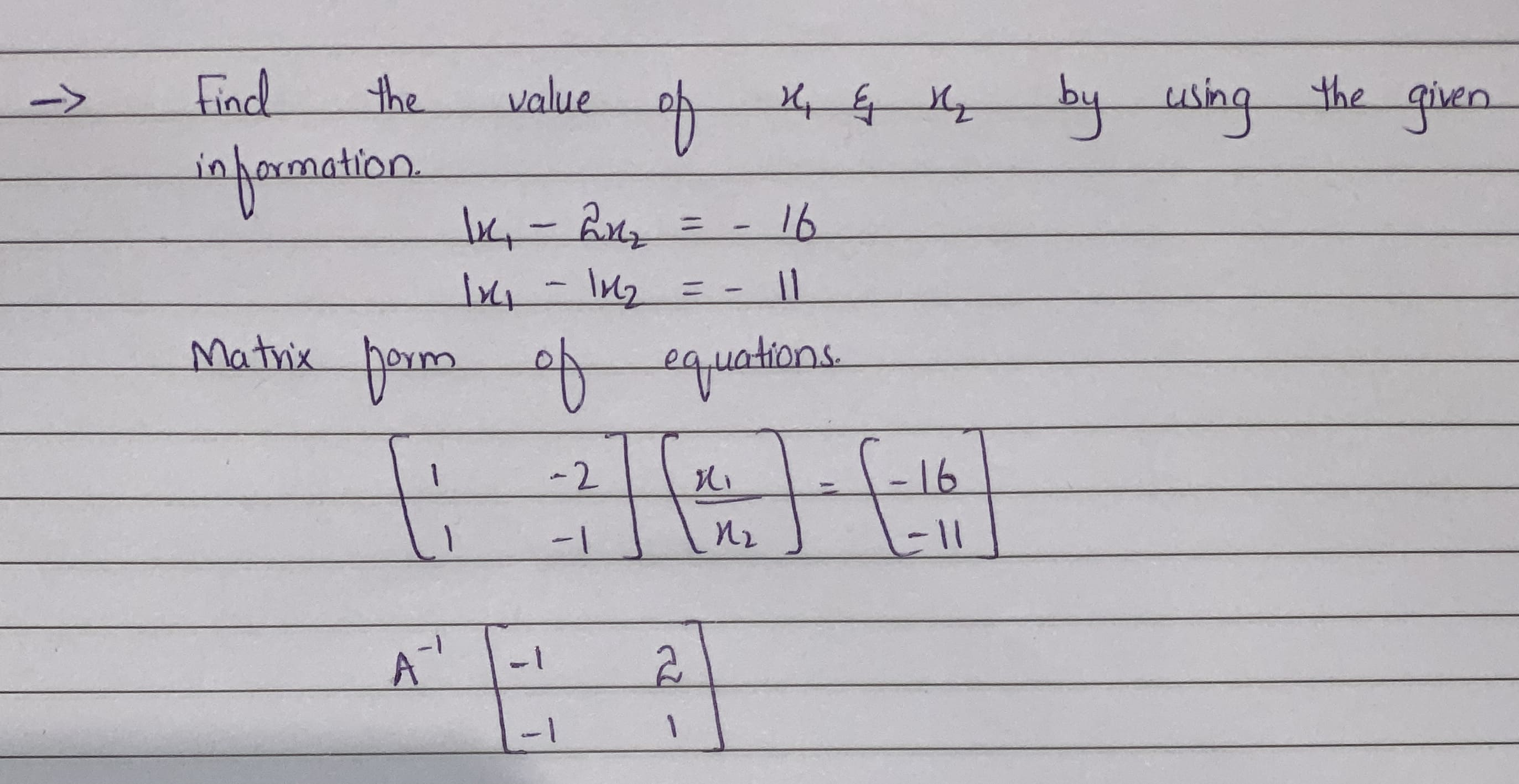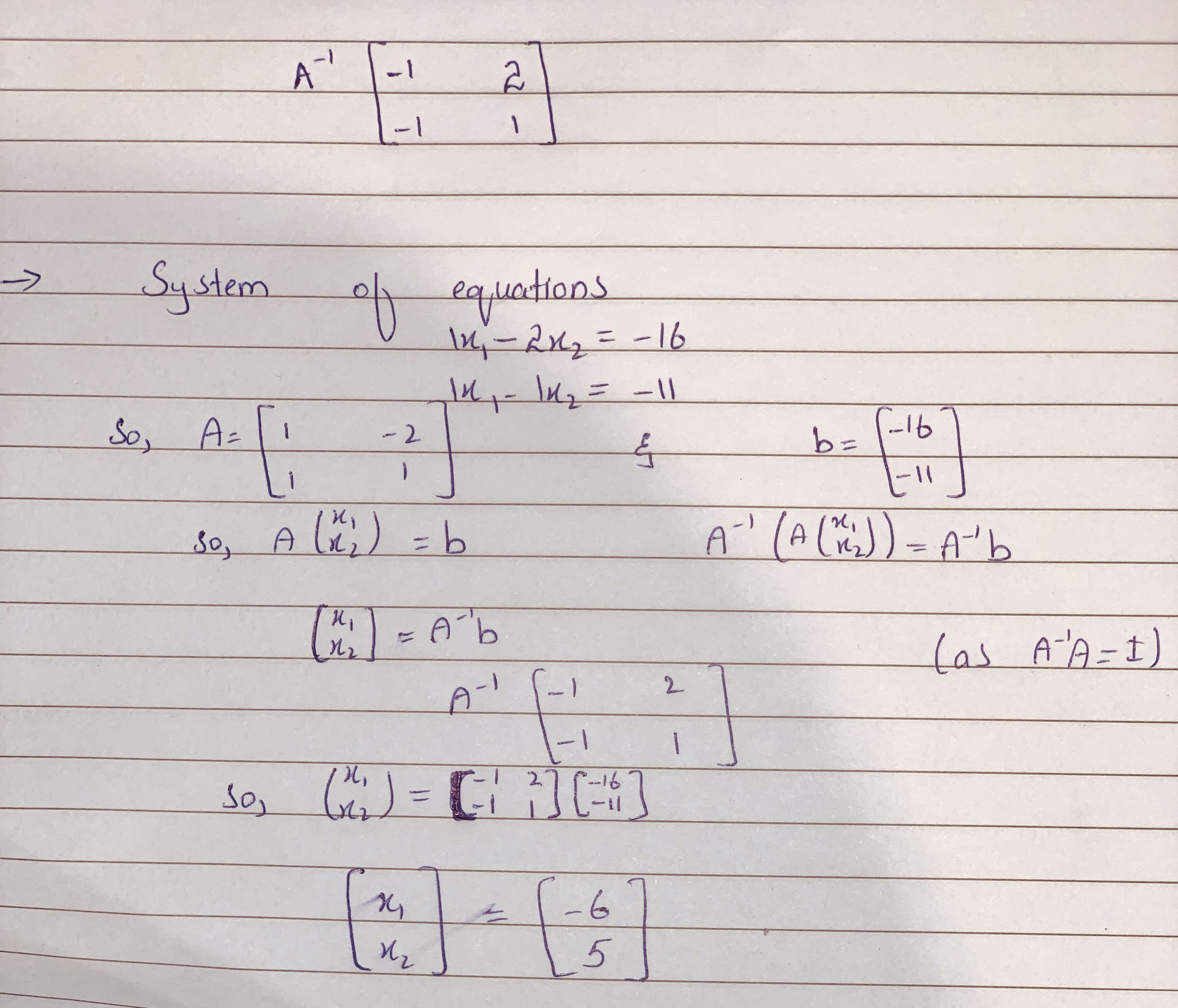#### Didn’t find what you are looking for?

Question# Find values of x_1 and x_2, which satisfy this system of equationbegin{cases}1x_1-2x_2=-161x_1-1x_2=-11end{cases}13310200941.JPG

Equations and inequalities
ANSWEREDFind values of $$\displaystyle{x}_{{1}}{\quad\text{and}\quad}{x}_{{2}}$$, which satisfy this system of equation
$$\begin{cases}1x_1-2x_2=-16\\1x_1-1x_2=-11\end{cases}$$